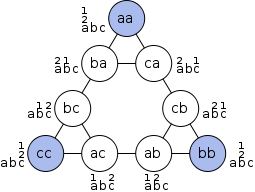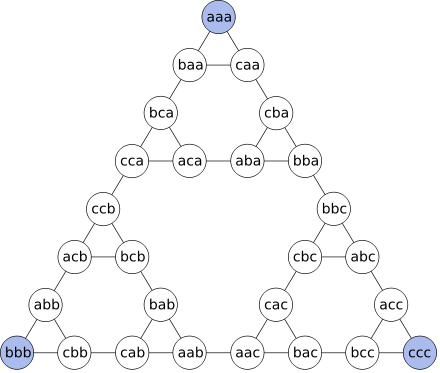## Graphical Representation

The game can be represented by an undirected graph, the nodes representing distributions of disks and the edges representing moves. For one disk, the graph is a triangle:The graph for 2 disks is 3 triangles arranged in a larger triangle:The nodes at the vertices of the outermost triangle represent distributions with all disks on the same peg.
For h+1 disks, take the graph of h disks and replace each small triangle with the graph for 2 disks.
For 3 disks the graph is:• call the pegs a, b and c
• list disk positions from left to right in order of increasing size

The sides of the outermost triangle represent the shortest ways of moving a tower from one peg to another one. The edge in the middle of the sides of the largest triangle represents a move of the largest disk. The edge in the middle of the sides of each next smaller triangle represents a move of each next smaller disk. The sides of the smallest triangles represent moves of the smallest disk.

In general, for a puzzle with n disks, there are 3n nodes in the graph; every node has three edges to other nodes, except the three corner nodes, which have two: it is always possible to move the smallest disk to the one of the two other pegs; and it is possible to move one disk between those two pegs except in the situation where all disks are stacked on one peg. The corner nodes represent the three cases where all the disks are stacked on one peg. The diagram for n + 1 disks is obtained by taking three copies of the n-disk diagram - each one representing all the states and moves of the smaller disks for one particular position of the new largest disk - and joining them at the corners with three new edges, representing the only three opportunities to move the largest disk. The resulting figure thus has 3^n+1 nodes and still has three corners remaining with only two edges.

As more disks are added, the graph representation of the game will resemble the Fractal figure, Sierpinski triangle. It is clear that the great majority of positions in the puzzle will never be reached when using the shortest possible solution; indeed, if the priests of the legend are using the longest possible solution (without re-visiting any position) it will take them 3^64 - 1 moves, or more than 10^23 years.